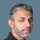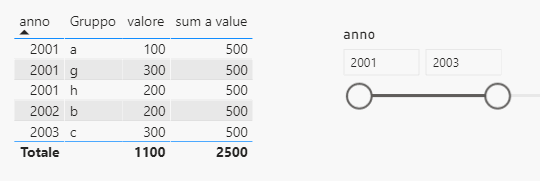cancel
Showing results for
Did you mean:Frequent Visitor

## Sum all value of one group keeping the filter of another table

Hello I have these 2 simple table

Tabella (2)CalendarioI would like to have report a table with one column that show the sum of all the value of the group "a" on all the row but only for the selected period

So If I filter only the year 2001-2003 I want all the row with the value 100. If I filter all the year I want all the row with the value 500 (100+400) like belowI have tryed some formulas like the below but noone of them give me the expected result

sum a value = calculate(sum('Tabella (2)'[valore]),CALCULATETABLE(ALLEXCEPT('Tabella (2)',calendario[anno]),'Tabella (2)'[Gruppo]="a"))

sum a value = calculate(sum('Tabella (2)'[valore]),ALLEXCEPT('Tabella (2)',calendario[anno]),'Tabella (2)'[Gruppo]="a")

sum a value = calculate(sum('Tabella (2)'[valore]),ALL('Tabella (2)'),'Tabella (2)'[Gruppo]="a")

The all function is deleting the filter on the year, and allexcept is not making the sum of all the "a" of the selected period

1 ACCEPTED SOLUTIONSuper User

@Guitarseb

I am not sure what results you need at grand total level, check this measure please:

``````sum a value =
CALCULATE(
SUM(Tabella2[valore]),
Tabella2[Gruppo] = "a",
ALLSELECTED(Tabella2)
)``````

________________________

If my answer was helpful, please consider Accept it as the solution to help the other members find it

Click on the Thumbs-Up icon if you like this reply 🙂

Did I answer your question? Mark my post as a solution! and hit thumbs up
4 REPLIES 4Frequent Visitor

Hello, i am trying to do the same thing here, but i can't fugure out how.. did you found a working solution eventually?Super User

@Guitarseb

I am not sure what results you need at grand total level, check this measure please:

``````sum a value =
CALCULATE(
SUM(Tabella2[valore]),
Tabella2[Gruppo] = "a",
ALLSELECTED(Tabella2)
)``````

________________________

If my answer was helpful, please consider Accept it as the solution to help the other members find it

Click on the Thumbs-Up icon if you like this reply 🙂

Did I answer your question? Mark my post as a solution! and hit thumbs upFrequent Visitor

Hi Funny, i found the problem. I was writing the formula in a new column instead of a new measure. So now your formula is working.

ThanksFrequent Visitor

No i'm sorry but I still get the same result. When I filter with the year the total is not filtered. I would like to filter all the data like the Subtotal at the end of the table and then filter only the group="a". This is the result I get with your formula: all 500 that is the sum of the value of the 2001 and 2006 (100+400)Announcements#### Exclusive opportunity for Women!

Join us for a free, hands-on Microsoft workshop led by women trainers for women where you will learn how to build a Dashboard in a Day!#### Power Platform Conference-Power BI and Fabric Sessions

Join us Oct 1 - 6 in Las Vegas for the Microsoft Power Platform Conference.Top Solution Authors
Top Kudoed Authors
Users online (3,392)